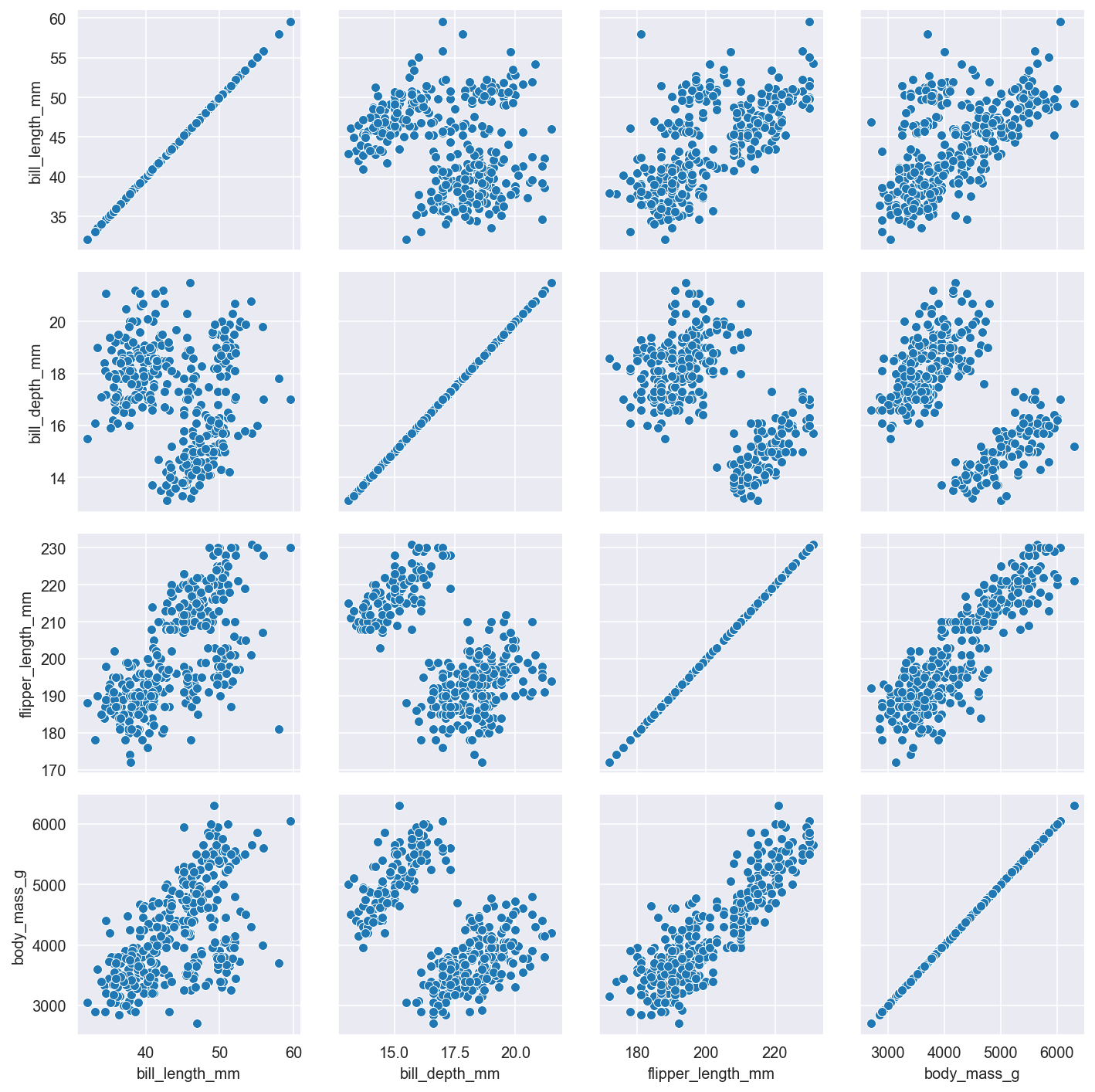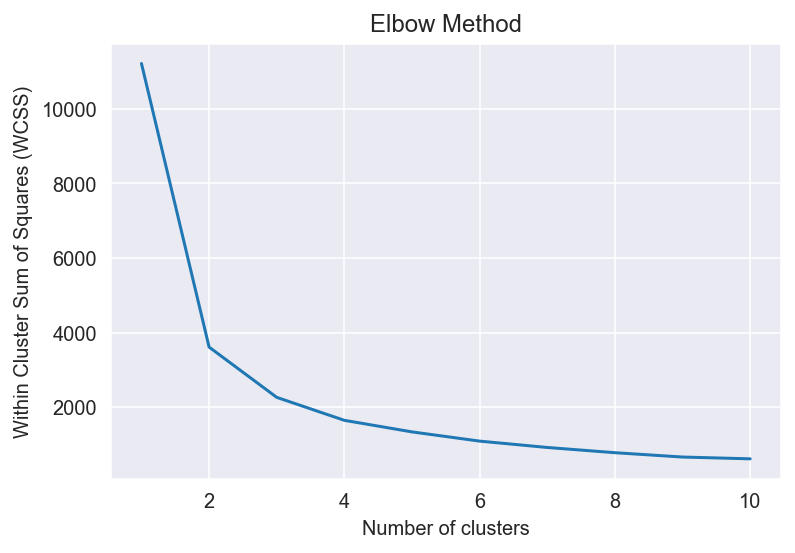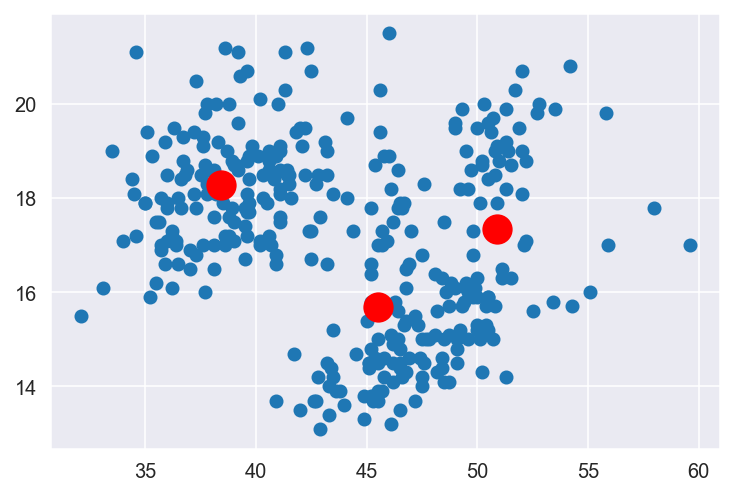Back to Templates

# Find and Visualize clusters with K-Means

This template helps you identify and visualize clusters in yourdata. The K Means algorithm aims to assign the data points in your dataset to K distinct clusters. After running the algorithm each observation (data point) will belong to the cluster whose center it is closest to.

```.css-y3yaiy{border:0;border-radius:4px;color:rgb(5, 25, 45);font-family:monospace;font-family:JetBrainsMonoNL;font-size:14px;font-variant-ligatures:none;letter-spacing:0;line-height:1.5;margin:0;padding:0;}```# Load packages
import pandas as pd
import seaborn as sns
import numpy as np
import matplotlib.pyplot as plt
from sklearn.cluster import KMeans
from sklearn.model_selection import train_test_split
sns.set_style('darkgrid')
%config InlineBackend.figure_format = 'retina'``````
``````# Upload your data as a csv file and load it as a data frame
speciesislandbill_length_mmbill_depth_mmflipper_length_mmbody_mass_gsex
``````# Visualize the correlation your data and identify variables for further analysis
g = sns.PairGrid(df)
g.map(sns.scatterplot);````````````X = np.array(df.loc[:,['bill_length_mm',                # Choose your variable names
'bill_depth_mm']])    \
.reshape(-1, 2)

# Determine optimal cluster number with elbow method
wcss = []

for i in range(1, 11):
model = KMeans(n_clusters = i,
init = 'k-means++',                 # Initialization method for kmeans
max_iter = 300,                     # Maximum number of iterations
n_init = 10,                        # Choose how often algorithm will run with different centroid
random_state = 0)                   # Choose random state for reproducibility
model.fit(X)
wcss.append(model.inertia_)

# Show Elbow plot
plt.plot(range(1, 11), wcss)
plt.title('Elbow Method')                               # Set plot title
plt.xlabel('Number of clusters')                        # Set x axis name
plt.ylabel('Within Cluster Sum of Squares (WCSS)')      # Set y axis name
plt.show()````````````kmeans = KMeans(n_clusters = 3,                 # Set amount of clusters
init = 'k-means++',             # Initialization method for kmeans
max_iter = 300,                 # Maximum number of iterations
n_init = 10,                    # Choose how often algorithm will run with different centroid
random_state = 0)               # Choose random state for reproducibility

pred_y = kmeans.fit_predict(X)

# Plot the data
plt.scatter(X[:,0],
X[:,1])

# Plot the clusters
plt.scatter(kmeans.cluster_centers_[:, 0],
kmeans.cluster_centers_[:, 1],
s=200,                             # Set centroid size
c='red')                           # Set centroid color
plt.show()``````### Find and Visualize clusters with K-Means

Identify and visualize clusters in your data.

Use Template